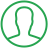# Counting Vowels In A String Python

Last Updated : Jul 1, 2023In this article we will show you the solution of counting vowels in a string python, the letter "a" "e" "I" "o" & "u" are all considered vowels. The remaining letters are all consonants (p, q, r, s, etc.).

A Python programme can accept user-supplied strings. Now let's discuss the concept of vowel going to count in string python.

## Step By Step Guide On Counting Vowels In A String Python :-

### Method - Using For loop and Lower() function

```str1 = input("Please enter the required string : ")
vowels = 0
str1.lower()
for i in str1:
if(i == 'a' or i == 'e' or i == 'i' or i == 'o' or i == 'u'):
vowels = vowels + 1
print("Total Number of Vowels in the String = ", vowels)
```
1. Here, you can see how we created a Python programme that uses the For loop & Lower() method to count vowel in a string.
2. At the start of the function, initialise the string that will take user input.
3. Then use the lower() function to convert the string to lowercase.
4. Then, using a for loop and an if statement, count the vowels in the string.
5. If Statement is being used inside of the For Loop to determine if the character is an a, e, I o, or u. If so, increase the value of the vowels; if not, skip that letter.
6. In order to determine how many vowels are present in this string overall, we use the print function at the end.

### Method - Using ASCII Value

```str1 = input("Please enter the own string : ")
vowels = 0
for i in str1:
if(ord(i) == 65 or ord(i) == 69 or ord(i) == 73
or ord(i) == 79 or ord(i) == 85
or ord(i) == 97 or ord(i) == 101 or ord(i) == 105
or ord(i) == 111 or ord(i) == 117):
vowels = vowels + 1
print("Total number of an vowel in this string = ", vowels)```
1. You can see that in this example, we have written Python code to count the amount of vowels in a text using ASCII values.
2. The string that receives user input is initialised at the beginning of the programme.
3. Using a for loop, an if statement, and the ord() function, count the vowels in the string after that.
4. By using the ord() method, the If Statement inside of the For Loop determines if the character is one of the following: a, e, I o, or u.
5. If so, increase the value of the vowels; if not, skip that letter.
6. In order to print the number of vowels, we utilise the print function at the end.

## Conclusion :-

Hence, we have successfully acquired the idea of vowel counting in a string of Python.

Vowels include the letters "a," "e," "I," "o," and "u." All of the letters that are left are consonant (p, q, r, s, etc.).

User-provided strings can be accepted by a Python programme.

I hope this article on counting vowels in a string python helps you and the steps and method mentioned above are easy to follow and implement.

##About Anjali

Experienced Computer Programmer with a broad range of experience in technology. Strengths in application development and Object Oriented architecture design, front end programming, usability and multimedia technology. Expert in coding languages such as C, C++ Java, JavaScript, PHP and more.